# Functional Geometry

Peter Henderson wrote an article about a method to describe pictures with functional programs. I've implemented it in Lisp. An updated version of the article uses curves and different shapes and has some references to other applications for this method, like "An Algebra of Music".

## How it works

The basic unit is a picture, which is a function, which must be called with three vectors: vector "a" for the offset from left bottom and vector "b" and "c" for the bounding box. The "grid" function returns such a picture function. For example we can define a simple figure:

```(defparameter *man*
(grid 14 20
(polygon
'((6 10) (0 10) (0 12) (6 12) (6 14)
(4 16) (4 18) (6 20) (8 20) (10 18)
(10 16) (8 14) (8 12) (10 12) (10 14)
(12 14) (12 10) (8 10) (8 8) (10 0)
(8 0) (7 4) (6 0) (4 0) (6 8)))))
```

Plotted it looks like this:Now we can define a function "beside", with the pictures "p" and "q" as arguments, which returns a function with three vectors as arguments, which calls the pictures "p" and "q" with this three vectors. So "p" and "q" are functions, which are arguments to a function, which in turn returns another function. This sounds complicated, but is very elegant, if once understanded. See the implementation section for how it is implemented.

If you use it, you simply write it like this:

```(beside *man* *man*)
```Some other functions:

```(above *man* *man*)
```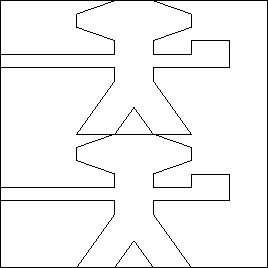```(rot *man*)
```But you'll get the full power of this concept, if you combine the functions to new functions. I never really understood, how it could be useful to use higher order functions as combinators, but it is immediatly clear, if you use it for geometry. For example we can define "quartet" like this:

```(defun quartet (p1 p2 p3 p4)
"returns the pictures p1-p4, layouted in a square"
(above (beside p1 p2) (beside p3 p4)))
```

You use it like this:

```(quartet *man* *man* *man* *man*)
```Another function:

```(cycle *man*)
```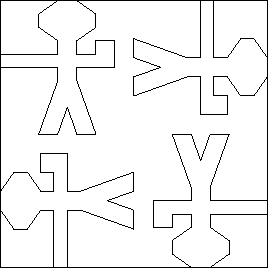## The fishes

With these functions you can define interesting graphics. These are the elements for the Escher drawing (see the article for details):

```;; defines part p of the fish
(defparameter *p*
(grid 16 16
'(((4 4) (6 0)) ((0 3)(3 4)) ((3 4)(0 8))
((0 8)(0 3)) ((4 5)(7 6)) ((7 6)(4 10))
((4 10)(4 5)) ((11 0)(10 4)) ((10 4)(8 8))
((8 8)(4 13)) ((4 13)(0 16)) ((11 0)(14 2))
((14 2)(16 2)) ((10 4)(13 5)) ((13 5)(16 4))
((9 6)(12 7)) ((12 7)(16 6)) ((8 8)(12 9))
((12 9)(16 8)) ((8 12)(16 10)) ((0 16)(6 15))
((6 15)(8 16)) ((8 16)(12 12)) ((12 12)(16 12))
((10 16)(12 14)) ((12 14)(16 13)) ((12 16)(13 15))
((13 15)(16 14)) ((14 16)(16 15)))))
```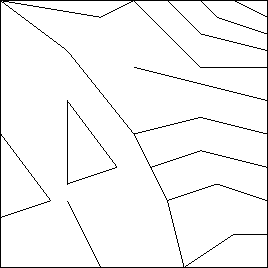```;; defines part q of the fish
(defparameter *q*
(grid 16 16
'(((2 0)(4 5)) ((4 5)(4 7)) ((4 0)(6 5))
((6 5)(6 7)) ((6 0)(8 5)) ((8 5)(8 8))
((8 0)(10 6)) ((10 6)(10 9)) ((10 0)(14 11))
((12 0)(13 4)) ((13 4)(16 8)) ((16 8)(15 10))
((15 10)(16 16)) ((16 16)(12 10)) ((12 10)(6 7))
((6 7)(4 7)) ((4 7)(0 8)) ((13 0)(16 6))
((14 0)(16 4)) ((15 0)(16 2)) ((0 10)(7 11))
((9 12)(10 10)) ((10 10)(12 12)) ((12 12)(9 12))
((8 15)(9 13)) ((9 13)(11 15)) ((11 15)(8 15))
((0 12)(3 13)) ((3 13)(7 15)) ((7 15)(8 16))
((2 16)(3 13)) ((4 16)(5 14)) ((6 16)(7 15)))))
``````;; defines part r of the fish
(defparameter *r*
(grid 16 16
'(((0 12)(1 14)) ((0 8)(2 12)) ((0 4)(5 10))
((0 0)(8 8)) ((1 1)(4 0)) ((2 2)(8 0))
((3 3)(8 2)) ((8 2)(12 0)) ((5 5)(12 3))
((12 3)(16 0)) ((0 16)(2 12)) ((2 12)(8 8))
((8 8)(14 6)) ((14 6)(16 4)) ((6 16)(11 10))
((11 10)(16 6)) ((11 16)(12 12)) ((12 12)(16 8))
((12 12)(16 16)) ((13 13)(16 10)) ((14 14)(16 12))
((15 15)(16 14)))))
```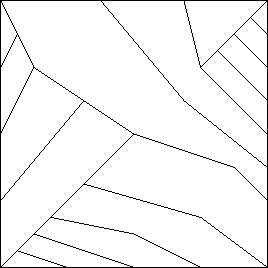```;; defines part s of the fish
(defparameter *s*
(grid 16 16
'(((0 0)(4 2)) ((4 2)(8 2)) ((8 2)(16 0))
((0 4)(2 1)) ((0 6)(7 4)) ((0 8)(8 6))
((0 10)(7 8)) ((0 12)(7 10)) ((0 14)(7 13))
((8 16)(7 13)) ((7 13)(7 8)) ((7 8)(8 6))
((8 6)(10 4)) ((10 4)(16 0)) ((10 16)(11 10))
((10 6)(12 4)) ((12 4)(12 7)) ((12 7)(10 6))
((13 7)(15 5)) ((15 5)(15 8)) ((15 8)(13 7))
((12 16)(13 13)) ((13 13)(15 9)) ((15 9)(16 8))
((13 13)(16 14)) ((14 11)(16 12)) ((15 9)(16 10)))))
``````(defparameter *t*
(quartet *p* *q* *r* *s*))
```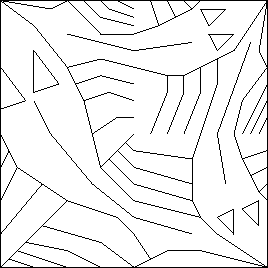```(defparameter *u*
(cycle (rot *q*)))
``````(defparameter *side1*
(quartet (blank) (blank) (rot *t*) *t*))
``````(defparameter *side2*
(quartet *side1* *side1* (rot *t*) *t*))
``````(defparameter *corner1*
(quartet (blank) (blank) (blank) *u*))
``````(defparameter *corner2*
(quartet *corner1* *side1* (rot *side1*) *u*))
``````(defparameter *pseudocorner*
(quartet *corner2* *side2* (rot *side2*) (rot *t*)))
``````(defparameter *fishes*
(cycle *pseudocorner*))
```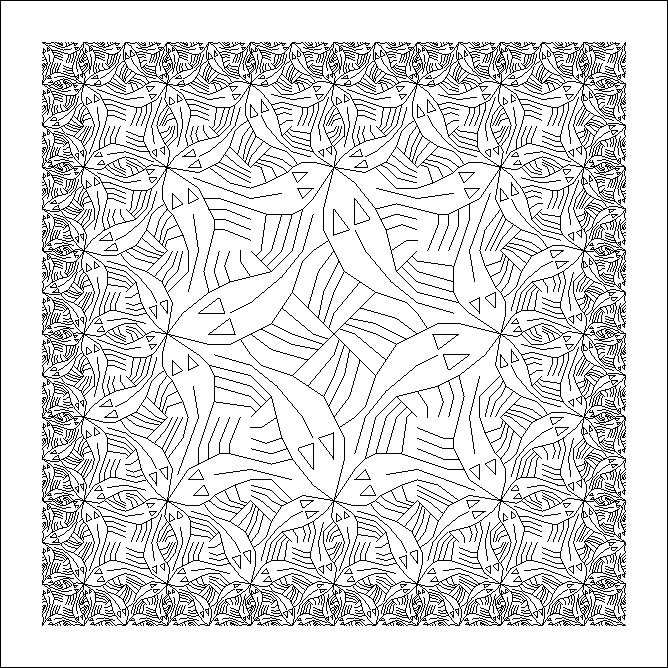Fishes in PDF and Postscript.

## The implementation

First we define some mathematical functions on 2D vectors, which are represented as a list of two numbers:

```(defun p* (vector m)
"vector scalar multiplication"
(destructuring-bind (vx vy) vector
(list (* vx m) (* vy m))))

(defun p/ (vector d)
"vector scalar division"
(destructuring-bind (vx vy) vector
(list (/ vx d) (/ vy d))))

(defun p+ (&rest vectors)
"#'+ for vectors"
(case (length vectors)
(0 '(0 0))
(1 (car vectors))
(otherwise (flet ((p+p (v1 v2)
(destructuring-bind (vx0 vy0) v1
(destructuring-bind (vx1 vy1) v2
(list (+ vx0 vx1) (+ vy0 vy1))))))
(reduce #'p+p vectors)))))

(defun p- (&rest vectors)
"#'- for vectors"
(case (length vectors)
(0 '(0 0))
(1 (p* (car vectors) -1))
(otherwise (flet ((p-p (v1 v2)
(destructuring-bind (vx0 vy0) v1
(destructuring-bind (vx1 vy1) v2
(list (- vx0 vx1) (- vy0 vy1))))))
(reduce #'p-p vectors)))))

```

And now the functions, with which we can construct pictures:

```(defun grid (m n s)
"defines a picture from lines in a grid"
(lambda (a b c)
(loop for line in s collect
(destructuring-bind ((x0 y0) (x1 y1)) line
(list (p+ (p/ (p* b x0) m) a (p/ (p* c y0) n))
(p+ (p/ (p* b x1) m) a (p/ (p* c y1) n)))))))

(defun polygon (points)
"converts the points, which specifies a polygon, in a list of lines"
(let ((start (car (last points))))
(loop for point in points collect
(list start point)
do (setf start point))))

(defun blank ()
"a blank picture"
(lambda (a b c)
(declare (ignore a b c))
'()))

(defun beside (p q)
"returns picture p besides picture q"
(lambda (a b c)
(let ((b-half (p/ b 2)))
(union (funcall p a b-half c)
(funcall q (p+ a b-half) b-half c)))))

(defun above (p q)
"returns picture q above picture p"
(lambda (a b c)
(let ((c-half (p/ c 2)))
(union (funcall p (p+ a c-half) b c-half)
(funcall q a b c-half)))))

(defun rot (p)
"returns picture p rotated by 90 degree"
(lambda (a b c)
(funcall p (p+ a b) c (p- b))))

(defun quartet (p1 p2 p3 p4)
"returns the pictures p1-p4, layouted in a square"
(above (beside p1 p2) (beside p3 p4)))

(defun cycle (p)
"returns four times the p, layouted in a square and rotated"
(quartet p (rot (rot (rot p))) (rot p) (rot (rot p))))
```

A platform dependent function shows a picture as Postscript:

```(defun plot (p)
" saves a picture as postscript and shows it"
(with-open-file (s "c:/tmp/test.ps"
:direction :output :if-exists :supersede)
(format s "500 500 scale~%")
(format s ".1 .1 translate~%")
(format s "0 setlinewidth~%")
(format s "0 0 moveto 1 0 lineto 1 1 lineto 0 1 lineto 0 0 lineto~%")
(dolist (line (funcall p '(0 0) '(1 0) '(0 1)))
(destructuring-bind ((x0 y0) (x1 y1)) line
(format s "~D ~D moveto ~D ~D lineto~%" (float x0) (float y0) (float x1) (float y1))))
(format s "stroke~%")
(format s "showpage~%"))
(sys:call-system "c:/gs/gs7.05/bin/gswin32.exe -g800x800 c:/tmp/test.ps"))
```

23. January 2005, Frank Buß